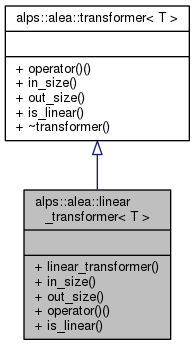ALPSCore reference
alps::alea::linear_transformer< T > Struct Template Reference

#include <transformer.hpp>

Inheritance diagram for alps::alea::linear_transformer< T >:Collaboration diagram for alps::alea::linear_transformer< T >:## Public Member Functions

template<typename Derived >
linear_transformer (const Eigen::MatrixBase< Derived > &mat)

size_t in_size () const

size_t out_size () const

column< T > operator() (const column< T > &in) const

bool is_linear () constPublic Member Functions inherited from alps::alea::transformer< T >
virtual ~transformer ()

## Detailed Description

### template<typename T> struct alps::alea::linear_transformer< T >

Linear transformation mediated by a matrix.

Definition at line 18 of file transformer.hpp.

## Constructor & Destructor Documentation

template<typename T >
template<typename Derived >
 alps::alea::linear_transformer< T >::linear_transformer ( const Eigen::MatrixBase< Derived > & mat )
inline

Definition at line 48 of file transformer.hpp.

## Member Function Documentation

template<typename T >
 size_t alps::alea::linear_transformer< T >::in_size ( ) const
inlinevirtual

expected number of components of the input vector

Implements alps::alea::transformer< T >.

Definition at line 52 of file transformer.hpp.

template<typename T >
 bool alps::alea::linear_transformer< T >::is_linear ( ) const
inlinevirtual

Guarantee transformation to be linear (allows certain optimizations)

Reimplemented from alps::alea::transformer< T >.

Definition at line 62 of file transformer.hpp.

template<typename T >
 column alps::alea::linear_transformer< T >::operator() ( const column< T > & in ) const
inlinevirtual

apply transformation

Implements alps::alea::transformer< T >.

Definition at line 56 of file transformer.hpp.

template<typename T >
 size_t alps::alea::linear_transformer< T >::out_size ( ) const
inlinevirtual

number of components of the returned vector

Implements alps::alea::transformer< T >.

Definition at line 54 of file transformer.hpp.

The documentation for this struct was generated from the following file: